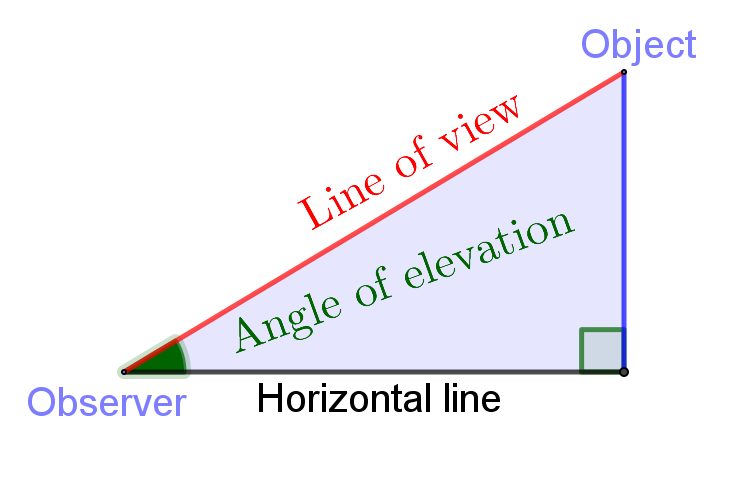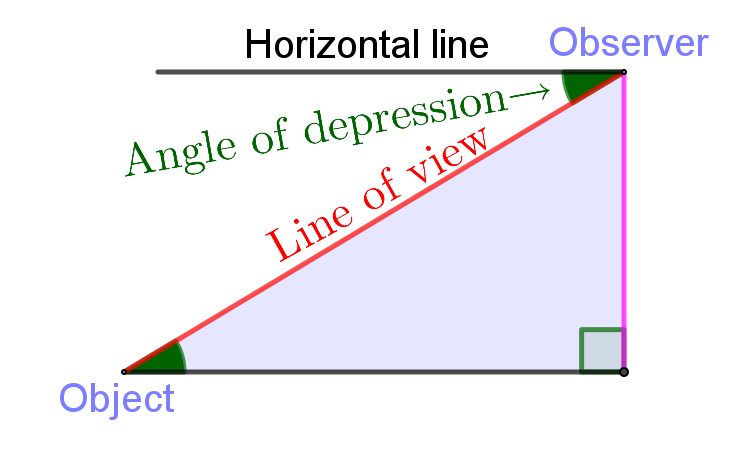# Height and Distance

## Introduction

Height and distance is a branch of trigonometry. Its main application is to find the height of any object like tree, building, mountain, pole, tower etc and the distance between two objects like distance between two edges of the river(i.e. width). This method is very useful in surveying, mapping map, and astronomy etc.

## Main points Related to Height and Distance

• Observer: A person who observes the object is known as observer.
• Object point: A point at which an observer observes is known as object point. If a man observes the top of a house, then man is an observer and the top of house is an object point.
• Line of view: Th straight line joining the observer and the object point is known as line of view.
• Horizontal line: Th straight line passing through observers eye and parallel to the ground ( plane surface ) is known as horizontal line.
• Angle of elevation: When an observer observes the object which is above the horizontal line, then the angle formed between horizontal line and line of view is known as angle of elevation.• Angle of depression: When an observer observes the object which is below the horizontal line, then the angle formed between horizontal line and line of view is known as angle of elevation.